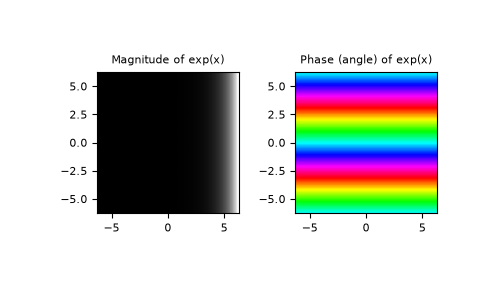numpy.trapz

numpy.expm1

# numpy.exp¶

numpy.exp(x, /, out=None, *, where=True, casting='same_kind', order='K', dtype=None, subok=True[, signature, extobj]) = <ufunc 'exp'>

Calculate the exponential of all elements in the input array.

Parameters: x : array_like Input values. out : ndarray, None, or tuple of ndarray and None, optional A location into which the result is stored. If provided, it must have a shape that the inputs broadcast to. If not provided or None, a freshly-allocated array is returned. A tuple (possible only as a keyword argument) must have length equal to the number of outputs. where : array_like, optional Values of True indicate to calculate the ufunc at that position, values of False indicate to leave the value in the output alone. **kwargs For other keyword-only arguments, see the ufunc docs. out : ndarray or scalar Output array, element-wise exponential of x. This is a scalar if x is a scalar.

See also

expm1
Calculate exp(x) - 1 for all elements in the array.
exp2
Calculate 2**x for all elements in the array.

Notes

The irrational number e is also known as Euler’s number. It is approximately 2.718281, and is the base of the natural logarithm, ln (this means that, if, then. For real input, exp(x) is always positive.

For complex arguments, x = a + ib, we can write. The first term,, is already known (it is the real argument, described above). The second term,, is, a function with magnitude 1 and a periodic phase.

References

  Wikipedia, “Exponential function”, https://en.wikipedia.org/wiki/Exponential_function
  M. Abramovitz and I. A. Stegun, “Handbook of Mathematical Functions with Formulas, Graphs, and Mathematical Tables,” Dover, 1964, p. 69, http://www.math.sfu.ca/~cbm/aands/page_69.htm

Examples

Plot the magnitude and phase of exp(x) in the complex plane:

>>> import matplotlib.pyplot as plt

>>> x = np.linspace(-2*np.pi, 2*np.pi, 100)
>>> xx = x + 1j * x[:, np.newaxis] # a + ib over complex plane
>>> out = np.exp(xx)

>>> plt.subplot(121)
>>> plt.imshow(np.abs(out),
...            extent=[-2*np.pi, 2*np.pi, -2*np.pi, 2*np.pi], cmap='gray')
>>> plt.title('Magnitude of exp(x)')

>>> plt.subplot(122)
>>> plt.imshow(np.angle(out),
...            extent=[-2*np.pi, 2*np.pi, -2*np.pi, 2*np.pi], cmap='hsv')
>>> plt.title('Phase (angle) of exp(x)')
>>> plt.show()